# Local statistics¶

## Introduction¶

This handout accompanies Chapter 8 in O’Sullivan and Unwin (2010).

## LISA¶

We compute some measures of local spatial autocorrelation.

First get the Auckland data.

```if (!require("rspat")) remotes::install_github("rspatial/rspat")
library(rspat)
auck <- spat_data("auctb")
```

Now compute the spatial weights. You can try other ways (e.g. `relation="touches"`)

```w <- relate(auck, relation="rook")
```

Compute the Getis Gi

```Gi <- autocor(auck\$TB, w, "Gi")
##   0.4241759  0.5623307  0.4047413  0.6819762 -1.3278352 -1.4086435
```

And make a map

```grays <- rev(gray(seq(0,1,.2)))
auck\$Gi <- Gi
plot(auck, "Gi", col=grays)
```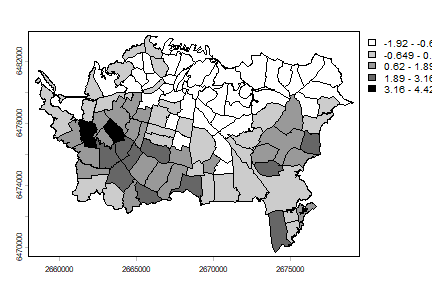Now on to the `Gi*` by including the focal area in the computation.

```#include "self"
diag(w) <- TRUE
auck\$Gistar <- autocor(auck\$TB, w, "Gi*")
plot(auck, "Gistar", main="Gi*", col=grays)
```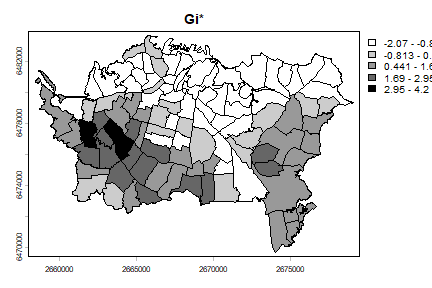This looks very similar to the local average, which we compute below.

```auck\$loc_mean <- apply(w, 1, function(i) mean(auck\$TB[i]))
plot(auck, "loc_mean", main="Local mean", col=grays)
```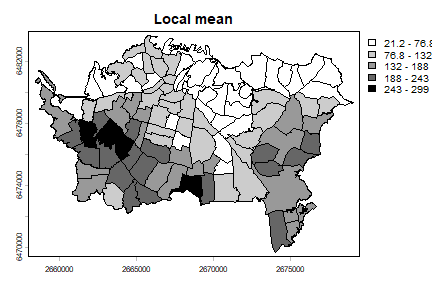The local Moran Ii has not been implemented in `terra`, but we can use `spdep` (a package that if focussed on these type of statistics and on spatial regression).

```library(spdep)
sfauck <- sf::st_as_sf(auck)
wr <- poly2nb(sfauck, row.names=sfauck\$Id, queen=FALSE)
lstw <- nb2listw(wr, style="B")
auck\$Ii <- localmoran(auck\$TB, lstw)
## Warning: [[[<-,SpatVector] only using the first column
plot(auck, "Ii", main="Local Moran", col=grays)
```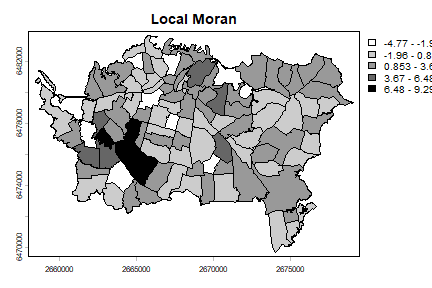In the above I followed the book by useing the raw count data. Howwever, it would be more approproate to use disease density instead. You can compute that like this:

```auck\$TBdens <- 10000 * auck\$TB / expanse(auck)
```

The 10000 is just to avoid very small numbers.

## Geographically weighted regression¶

Here is an example of GWR with California precipitation data.

```cts <- spat_data("counties")
p <- spat_data("precipitation")
##      ID                 NAME   LAT    LONG ALT  JAN FEB MAR APR MAY JUN JUL
## 1 ID741         DEATH VALLEY 36.47 -116.87 -59  7.4 9.5 7.5 3.4 1.7 1.0 3.7
## 2 ID743  THERMAL/FAA AIRPORT 33.63 -116.17 -34  9.2 6.9 7.9 1.8 1.6 0.4 1.9
## 3 ID744          BRAWLEY 2SW 32.96 -115.55 -31 11.3 8.3 7.6 2.0 0.8 0.1 1.9
## 4 ID753 IMPERIAL/FAA AIRPORT 32.83 -115.57 -18 10.6 7.0 6.1 2.5 0.2 0.0 2.4
## 5 ID754               NILAND 33.28 -115.51 -18  9.0 8.0 9.0 3.0 0.0 1.0 8.0
## 6 ID758        EL CENTRO/NAF 32.82 -115.67 -13  9.8 1.6 3.7 3.0 0.4 0.0 3.0
##    AUG SEP OCT NOV DEC
## 1  2.8 4.3 2.2 4.7 3.9
## 2  3.4 5.3 2.0 6.3 5.5
## 3  9.2 6.5 5.0 4.8 9.7
## 4  2.6 8.3 5.4 7.7 7.3
## 5  9.0 7.0 8.0 7.0 9.0
## 6 10.8 0.2 0.0 3.3 1.4
plot(cts)
points(p[,c("LONG", "LAT")], col="red", pch=20)
```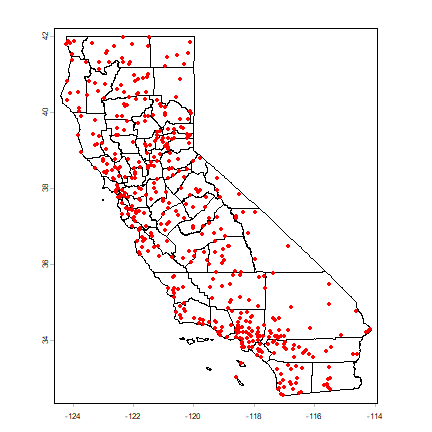Compute annual average precipitation

```p\$pan <- rowSums(p[,6:17])
```

Global regression model

```m <- lm(pan ~ ALT, data=p)
m
##
## Call:
## lm(formula = pan ~ ALT, data = p)
##
## Coefficients:
## (Intercept)          ALT
##      523.60         0.17
```

Create `sf` objects with a planar crs.

```alb <- "+proj=aea +lat_1=34 +lat_2=40.5 +lat_0=0 +lon_0=-120 +x_0=0 +y_0=-4000000 +datum=WGS84"
sp <- vect(p, c("LONG", "LAT"), crs="+proj=longlat +datum=NAD83")
sp <- terra::project(sp, alb)
spsf <- sf::st_as_sf(sp)
vctst <- terra::project(cts, alb)
ctst <- sf::st_as_sf(vctst)
```

Get the optimal bandwidth

```library( spgwr )
## NOTE: This package does not constitute approval of GWR
## as a method of spatial analysis; see example(gwr)
bw <- gwr.sel(pan ~ ALT, crds(sp), data=spsf)
## Bandwidth: 526221.2 CV score: 64886877
## Bandwidth: 850593.7 CV score: 74209069
## Bandwidth: 325747.9 CV score: 54001111
## Bandwidth: 201848.7 CV score: 44611207
## Bandwidth: 125274.7 CV score: 35746317
## Bandwidth: 77949.4 CV score: 29181734
## Bandwidth: 48700.75 CV score: 22737206
## Bandwidth: 30624.09 CV score: 17457182
## Bandwidth: 19452.1 CV score: 15163453
## Bandwidth: 12547.43 CV score: 19452224
## Bandwidth: 22792.75 CV score: 15513008
## Bandwidth: 17052.64 CV score: 15709986
## Bandwidth: 20218.98 CV score: 15167455
## Bandwidth: 19767.99 CV score: 15156930
## Bandwidth: 19790.05 CV score: 15156924
## Bandwidth: 19781.39 CV score: 15156920
## Bandwidth: 19781.48 CV score: 15156920
## Bandwidth: 19781.47 CV score: 15156920
## Bandwidth: 19781.47 CV score: 15156920
## Bandwidth: 19781.47 CV score: 15156920
## Bandwidth: 19781.47 CV score: 15156920
bw
##  19781.47
```

Create a regular set of points to estimate parameters for.

```r <- rast(vctst, res=10000)
r <- rasterize(vect(ctst), r)
newpts <- geom(as.points(r))[, c("x", "y")]
```

Run the `gwr` function

```g <- gwr(pan ~ ALT, crds(sp), data=spsf, bandwidth=bw, fit.points=newpts[, 1:2])
g
## Call:
## gwr(formula = pan ~ ALT, data = spsf, coords = crds(sp), bandwidth = bw,
##     fit.points = newpts[, 1:2])
## Kernel function: gwr.Gauss
## Fixed bandwidth: 19781.47
## Fit points: 4090
## Summary of GWR coefficient estimates at fit points:
##                     Min.     1st Qu.      Median     3rd Qu.      Max.
## X.Intercept. -846.293016   77.986323  328.578941  729.597303 3452.1971
## ALT            -3.961744    0.034145    0.201568    0.418714    4.6022
```

Link the results back to the raster.

```slope <- intercept <- r
slope[!is.na(slope)] <- g\$SDF\$ALT
intercept[!is.na(intercept)] <- g\$SDF\$"(Intercept)"
s <- c(intercept, slope)
names(s) <- c("intercept", "slope")
plot(s)
```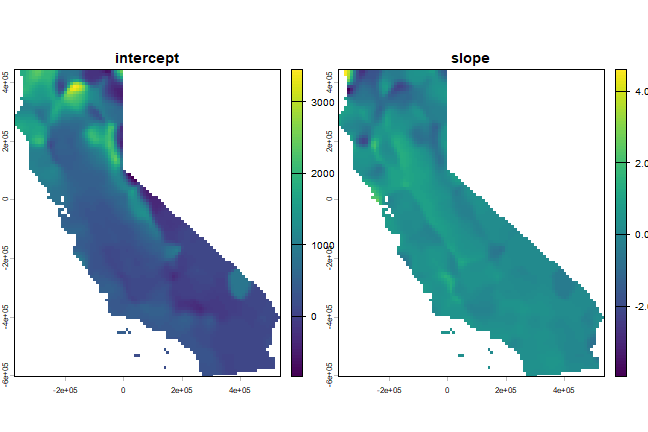See this page for a more detailed example of geographically weighted regression.